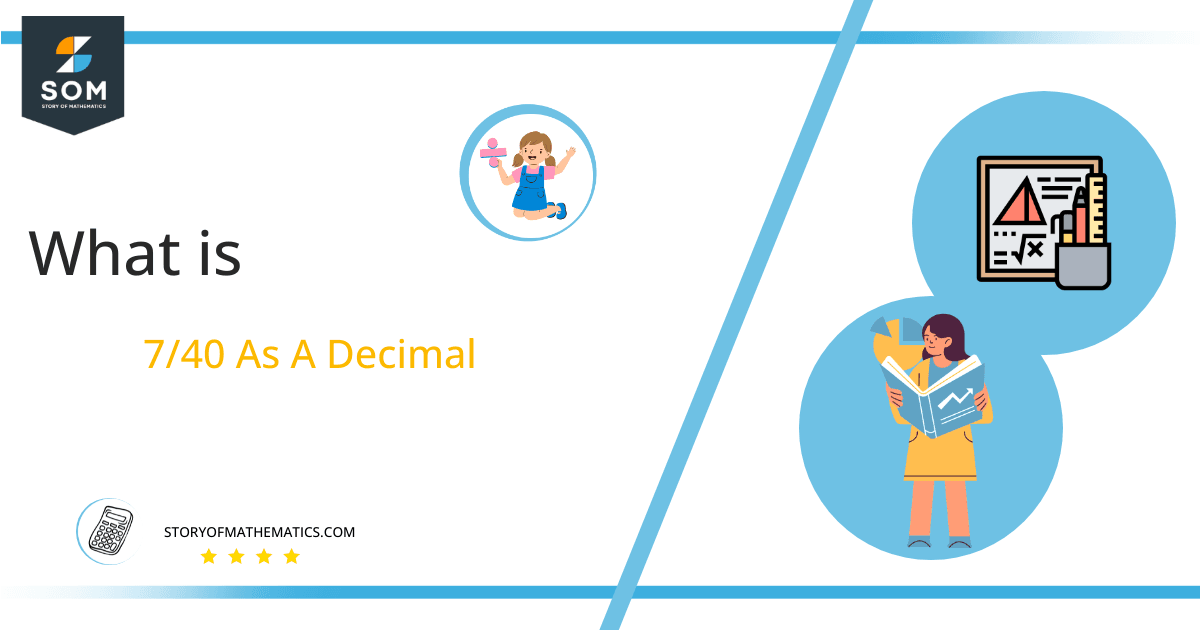# What Is 7/40 as a Decimal + Solution With Free Steps

The fraction 7/40 as a decimal is equal to 0.175.

The fraction 7/40 can be represented as a decimal. In decimal, the first part is a whole number whereas the second part represents the remaining fraction. As the numerator is less than the denominator in the fraction 7/40, therefore it is a proper fraction.

Here, we are interested more in the types of division that results in a Decimal value, as this can be expressed as a Fraction. We see fractions as a way of showing two numbers having the operation of Division between them that result in a value that lies between two Integers.Now, we introduce the method used to solve said fraction to decimal conversion, called Long Division which we will discuss in detail moving forward. So, let’s go through the Solution of fraction 7/40.

## Solution

First, we convert the fraction components i.e., the numerator and the denominator, and transform them into the division constituents i.e., the Dividend and the Divisor respectively.

This can be seen done as follows:

Dividend = 7

Divisor = 40

Now, we introduce the most important quantity in our process of division, this is the Quotient. The value represents the Solution to our division, and can be expressed as having the following relationship with the Division constituents:

Quotient = Dividend $\div$ Divisor = 7 $\div$ 40

This is when we go through the Long Division solution to our problem. The long division process for the above fraction is shown in figure 1.Figure 1

## 7/40 Long Division Method

We start solving a problem using the Long Division Method by first taking apart the division’s components and comparing them. As we have 7, and 40 we can see how 7 is Smaller than 40, and to solve this division we require that 7 be Bigger than 40.

This is done by multiplying the dividend by 10 and checking whether it is bigger than the divisor or not. And if it is then we calculate the Multiple of the divisor which is closest to the dividend and subtract it from the Dividend. This produces the Remainder which we then use as the dividend later.

Now, we begin solving for our dividend 7, which after getting multiplied by 10 becomes 70.

We take this 70 and divide it by 40, this can be seen done as follows:

70 $\div$ 40 $\approx$ 1

Where:

40 x 1 = 40

This will lead to the generation of a Remainder equal to 70 – 40 = 30, now this means we have to repeat the process by Converting the 30 into 300 and solving for that:

300 $\div$ 40 $\approx$ 7

Where:

40 x 7 = 280

This, therefore, produces another remainder which is equal to 300 – 280 = 20. Now we must solve this problem to Third Decimal Place for accuracy, so we repeat the process with dividend 200.

200 $\div$ 40 = 5

Where:

40 x 5 = 200

Finally, we have a Quotient generated after combining the three pieces of it as 0.175, with a Remainder equal to 0.Images/mathematical drawings are created with GeoGebra.